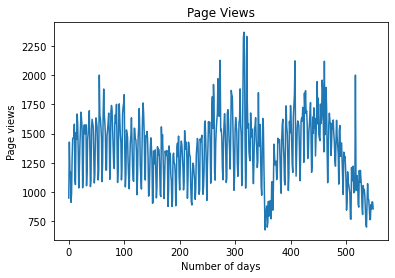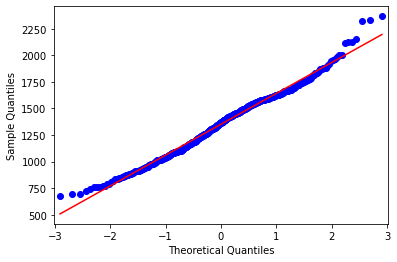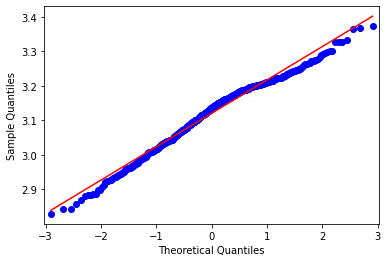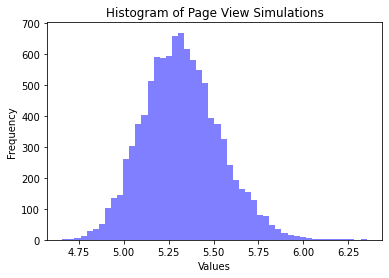蒙特卡洛方法分析Web页面浏览量概率分布>>> series = value;
>>> skewness = series.skew();
>>> print("Skewness:");
>>> print(round(skewness,2));
Skewness:
0.17

QQ图表明，除了出现的异常值外，大多数值的分布都是正态分布。>>> mu=np.mean(logvalue)
>>> sigma=np.std(logvalue)
>>> x = mu + sigma * np.random.lognormal(mu, sigma, 10000)
>>> num_bins = 50>>> logvalue=pd.Series(logvalue)
>>> logseries = logvalue;
>>> skewness = logseries.skew();
>>> print("Skewness:");
>>> print(round(skewness,2));
Skewness:
-0.41蒙特卡罗模拟

>>> mu=np.mean(logvalue)
>>> sigma=np.std(logvalue)
>>> x = mu + sigma * np.random.lognormal(mu, sigma, 10000)
>>> num_bins = 50

array([5.21777304, 5.58552424, 5.39748092, ..., 5.27737933, 5.42742056, 5.52693816])• 1）大多数网页浏览量的范围；
• 2）分布中极值的范围。

结论

• 蒙特卡罗模拟的应用

• 偏态在定义分布中的作用

• 如何进行模拟以识别获得极值的概率

http://panchuang.net/

sklearn机器学习中文官方文档：
http://sklearn123.com/

http://docs.panchuang.net/